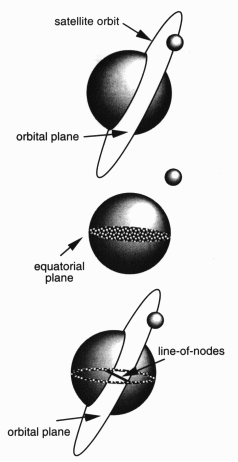A# line of nodesA line of nodes is a line that runs through the points of intersection between the orbits of two celestial bodies. In other words, it is the line that connects the ascending node and descending node of the orbit of one body as it intersects the orbit of another body.

In the context of celestial mechanics, the ascending node is the point where an object's orbit crosses the plane of reference from south to north; the descending node is the point where the orbit crosses the plane of reference from north to south. The line of nodes is the line that connects these two points and is often used as a reference in the study of the orbits of celestial bodies. The reference plane, usually the ecliptic or, in the case of a satellite, Earth's equatorial plane.

The line of nodes is important in the study of celestial mechanics because it is used to determine the relative positions of celestial bodies in their orbits. For example, the angle between the line of nodes and the reference plane can be used to determine the inclination of an object's orbit. Additionally, the position of the line of nodes in the orbit can be used to determine the longitude of the ascending node, which is an important parameter in the study of the dynamics of planetary systems.